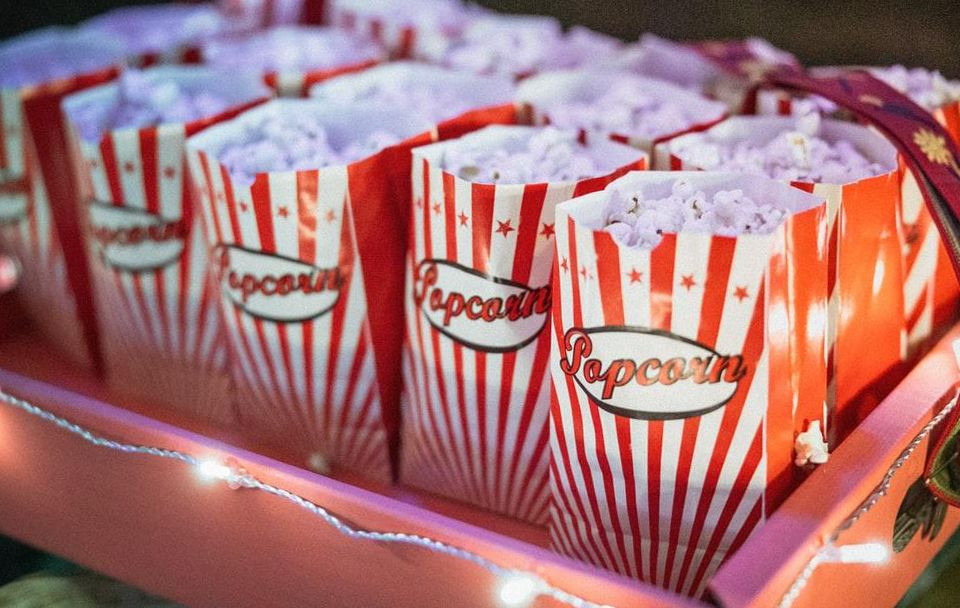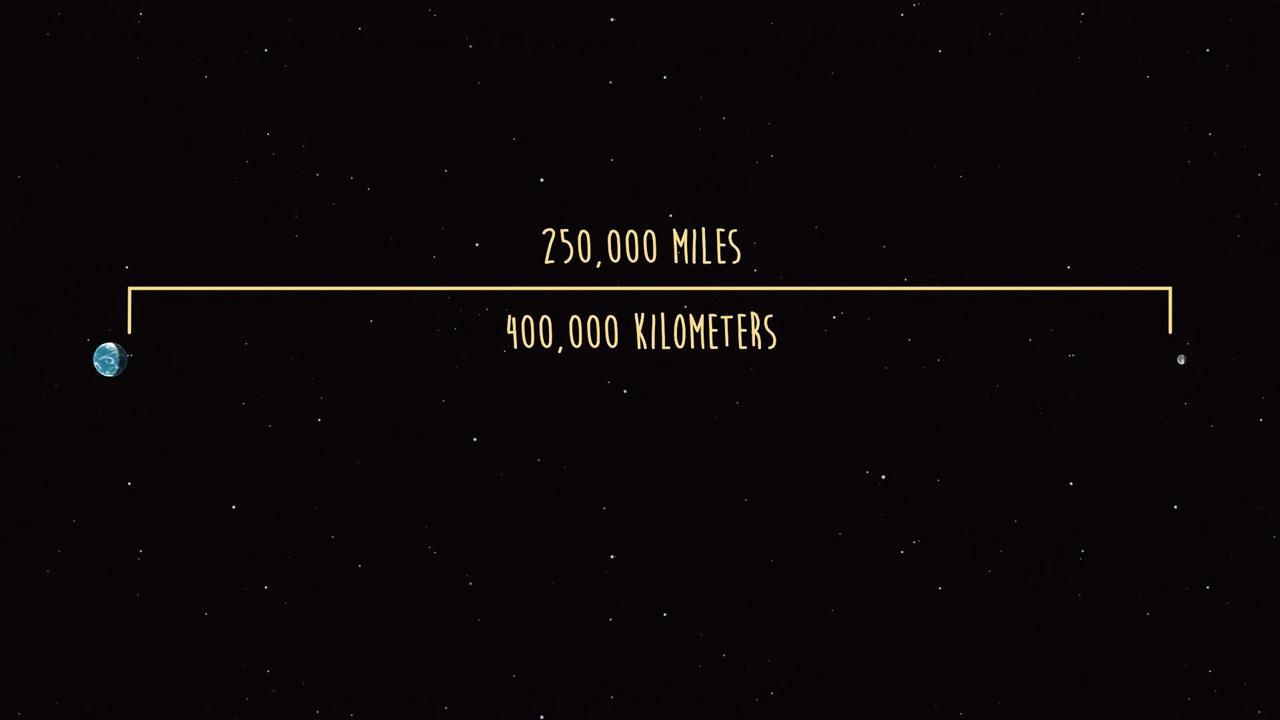# The Ways We Use Math in the Real World: 8 Examples

ParentingNo wonder many children ask themselves: “How will those abstract equations help me in life? What’s the point of practicing ‘John bought 45 watermelons’ equations all the time if nothing of this happens in real life?”

Well, it does happen, but the problem is that sometimes schools can’t deliver this knowledge in the right way. Teaching children math through real-life world problems is a whole different story.

## How Is Math Used in the Real World?

For many people, math doesn’t have any practical application. After all, most of us leave all those equations behind after we graduate from school.

But the truth is that we use math concepts in our daily lives without realizing it. Going shopping, cooking, working – these and many other activities touch on numerical calculations.

Being fluent in math means being successful in thousands of activities. Math develops logical thinking and spatial skills. It also helps your brain recruit certain areas better.

However, if you look at math on a broader scale, you will notice that human civilization as we know it appeared thanks to ancient Greek mathematics. Everything from architecture and machinery to medicine and iPads exist thanks to calculations.

Math for Kids

Is Your Child Struggling With Math?
1:1 Online Math Tutoring## Math in the Real World: 4 Examples

Teachers often bring real-life situations to the classrooms to make math more sensible and contextualized. Check these real-world math problems:

### #1 Which Popcorn Container Is Better?You go to the cinema anticipating getting a big popcorn bag to make your mouth busy for the rest of the movie. But then you step to the popcorn stand and see two popcorn bags, a coned one and a cylindrical one. You can get any for \$5, and both look equally big.

Which one should you choose? At first sight, there is no difference. But mathematicians know you will pay a higher price for a coned bag.

The cone’s volume is always 1/3 of the cylinder’s. As a result, the cylindrical bag will fit more popcorn, which means you will get more snacks for the same price.

### #2 Why Do Bees Construct Hexagons in Their Hives?Bees seem to be some of the most hardworking living beings, but these little buzzy things are also practical about space allocation. They never use any shape but a hexagon for their hives.

The question is: Why not round honeycombs? Round cells will not fit well because they will leave huge gaps. Then, why not triangle or square cells? They won’t be efficient either.

A lot of math happens in those hexagon cells. The hexagon has the smallest perimeter for a given surface area.

Let’s assume the hexagon’s sides are 5 cm long. The formula of a perimeter is the sum of all sides: 5 x 6 = 30. So, the hexagon with 5-cm sides has a perimeter of 30 cm.

The hexagon’s surface area formula is: (3 × √3 × S2) ÷ 2, where “S” is the length of the hexagon’s side. For our hexagon, we have the following formula: (3 × √3 × 25) ÷ 2 = 64.95 cm2.

Let’s do the same to a triangle with a perimeter of 30 cm. It will have 10 cm sides and a surface area of only 43.3 cm2.

If you want to build a triangle with a surface area of 64.95 cm2, it will have a perimeter of 37.75 cm, 25% longer than that of a hexagon.

So, if bees built triangular cells, they would have to use 25% more wax to match the hexagon’s surface area.

Moreover, the hexagon is the strongest shape for honeycomb walls as it has six stiffeners that transfer physical load more evenly than a triangle or a square.

### #3 Folding Paper Problem

Do you know that paper folding has a fascinating mathematical background? You must have heard that you can’t fold an A4 sheet of paper more than eight times.

The current world record is 12 times, but it worked only with a 1.219 km-long paper sheet. You may have also heard that folding paper 42 times will make it as thick as the distance to the Moon. It’s because its thickness grows exponentially with each fold. Let’s assume an A4 paper sheet is 0.1 mm thick. Here is how thick it will be with each fold:

• No fold – 0.1 mm thickness.
• One fold = 2 times thickness. There are two layers of paper. The total thickness is 0.2 mm.
• Two folds = 2². You will get four layers because you double the first two layers while folding.
• Three folds = 2³. You will double four layers. There will be eight layers.
• Four folds = 2⁴. You will double eight layers, totaling 16.
• Five folds = 2⁵. Doubling 16 results in 32.
• Six folds = 2⁶. Doubling 32 equals 64.
• Seven folds = 2⁷. Doubling 64 equals 128.

As you see, a paper sheet folded seven times has 128 layers. It will become 12.8 mm thick (128 x 0.1 = 12.8 mm). At this point, the paper becomes too strong and has too little surface area left for further folding.

#### Folding Paper to the Moon

What about 42 folds? They will result in 4,398,046,511,104 or 4.39 trillion layers. The paper thickness will theoretically reach 439.8 billion millimeters or 439.8 million meters. In its turn, 439.8 million meters equals 439,804 kilometers, while the distance between the Earth and the Moon is 384,400 kilometers.

So, mathematically speaking, you can fold the paper to the point when its height will reach the Moon and beyond.

But the problem is that with each fold, the visible surface area of the paper decreases by the scale factor of ½ (the real surface area doesn’t decrease). In other words, the paper becomes 50% of its original size with the first fold. Then, it will become half of the size of the first fold.

If you fold it 42 times, the visible surface area will be smaller than the atom. Thus, you can’t physically make it that small. So, this is what happens behind a simple “you can’t fold a paper more than seven times,” and it’s one of the direct examples of how math is used in the real world.### #4 Becoming Rich with Geometric Progression

School money topics often lead to many jokes about math. For instance, math is the only place where people casually buy 49 watermelons, and no one asks why.

You can make a money topic much more relatable to children if you start talking about fortune and wealth using a simple interest example – the stock market and interest rates.

If you put \$1,000 in banks and stocks, how much will you get in return in several years?

The prices of different stocks grow, and sometimes \$1,000 can become \$10,000 or even \$100,000 over the years, increasing in geometric progression.

But that’s a single-time investment. What about putting money into stocks and banks every month?

Another example is the crypto market where these processes speed up by many factors. Sometimes, lucky investors become millionaires overnight due to the high price fluctuations of different crypto assets.

These examples show children how math works in real life and enhance their financial literacy. This practice gives them answers to some of the most relatable questions: How to get off the rat race? How to become independent?

Math for Kids

Is Your Child Struggling With Math?
1:1 Online Math Tutoring## 4 Ways to Use Math in the Real World

Connecting math to real life will become easy with these activities:

### #1 Measurements

Many jobs require measurements, from construction and post to meteorology. You can show your kid that measurements matter if they want to send a package, know whether their Playstation fits their table, etc.

So, buy them a measuring tape and let them measure something. Be smart and incentivize your kids to do these tasks. For instance, ask them for help with sending a package to grandparents.

Let them measure the weight and dimensions of the shipping box or ask to keep a temperature diary by using a thermometer.

### #2 Practicing Percentages while Searching for Discounts

If your child doesn’t feel easy about percentages, introduce them to Amazon or eBay. Online shopping is the best way to learn percentages due to limitless percentage discounts here and there.

Their favorite shoes cost \$100. How much can they save using a 20% sign-in discount? Such incentives will motivate young minds to become fluent in percentages.

Sometimes, calculating the percentage of a number is as easy as multiplying it. For instance, to know how much is 20% of 5, one can multiply 5 by 0.20.

### #3 Cooking

Little kids may wonder how much math happens in the kitchen. So, allow your child to get closer with kitchen math: three pounds of tomatoes, four ounces of ice cream, etc. One teaspoon of ground coffee or ½ ounce of cinnamon? How much is the 1:1 proportion?

All cooking recipes require counting and measurement skills, and it’s an excellent chance to connect math to real life. Cooking develops understanding of ratios, proportions, and fundamental arithmetic operations.

### #4 Time Management

The concept of time challenges many students. However, it’s an essential skill a child has to get used to from a young age.

By practicing time management skills, children will learn how to tell time on the clock and manage their assignments better. Time management involves the following skills:

• Analog and digital clock time telling.
• Skip counting by five.
• Counting to 60.
• Time concepts like half past, quarter past, quarter to, etc.
• Logic reasoning and planning skills.

## The Bottom Line

Presentation of math topics through relatable examples helps children understand how valuable this subject is. This approach has a much higher chance of sparking children’s interest in mathematics.

At Brighterly, tutors apply play-based learning approaches to help children study math with fun. If your kid needs more math practice, you can enroll them in one of Brighterly’s online math courses. Children will enjoy sophisticated math theory taught in simple words with real-world math problems worksheets and other fun exercises that relate to real life.

Math Catch Up Program• Learn Math Simple - Fast - Effective
• Overcome math obstacles and reach new heights with Brighterly.

Simple - Fast - Effective
Overcome math obstacles and reach new heights with Brighterly.